# 3.5 Coercivity reduction in FePt/FeRh film: necessity of multiscale modelingWe will study the switching properties of a soft/hard bilayer film using two different models. The first will be a standard micromagnetic model and the second the multiscale modeling already introduced in Section 2.2.4. From their comparison with the previous results of a 1-D model by K. Guslienko et al. [Guslienko 04], which will be referred in this section as 1D'' model, we will show the necessity of multiscale modeling in the case of imperfect interfaces. The system modeled, represented in Fig. 3.15, has thicknesses of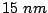of FePt and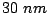of FeRh and the in-plane dimensions are. Periodic boundary conditions in the magnetostatic potential as well as in the exchange interaction are used in x,y directions, but not in z direction where free boundary conditions are used. Due to the system configuration and the periodic boundary conditions, the magnetostatic interaction yields an in-plane shape anisotropy equivalent to that of an infinite film. The temperature is supposed to be higher than the transition temperature, therefore, the FeRh is in its ferromagnetic state. The magnetocrystalline anisotropy of the FeRh was neglected and due to its large saturation magnetization the shape anisotropy is predominant. The easy axis of the FePt was parallel to the z direction, normal to the film plane. The following value of the material constants were used:and.

In the micromagnetic simulations, as well as in the micromagnetic zones in the multiscale model, we used the discretization length of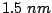(smaller than the domain wall width in FePt of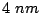), therefore, the total number of cells in the film is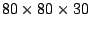. The validity of our micromagnetic simulations depends on the correct domain wall description. We have checked that the numerically calculated domain wall profile in pure FePt has the correct width in comparison to the theoretical prediction. In Fig. 3.16 we present a comparison between a perpendicular exchange spring (the dependence) calculated within 1-D atomistic approach and 3-D micromagnetic simulations when the exchange between the two layers has almost the bulk value. Although the two models give slightly different coercive fields, for applied fields close to the switching field the domain walls are almost identical. Therefore, our micromagnetic approach correctly describes the domain wall formation in this (strongly coupled) case.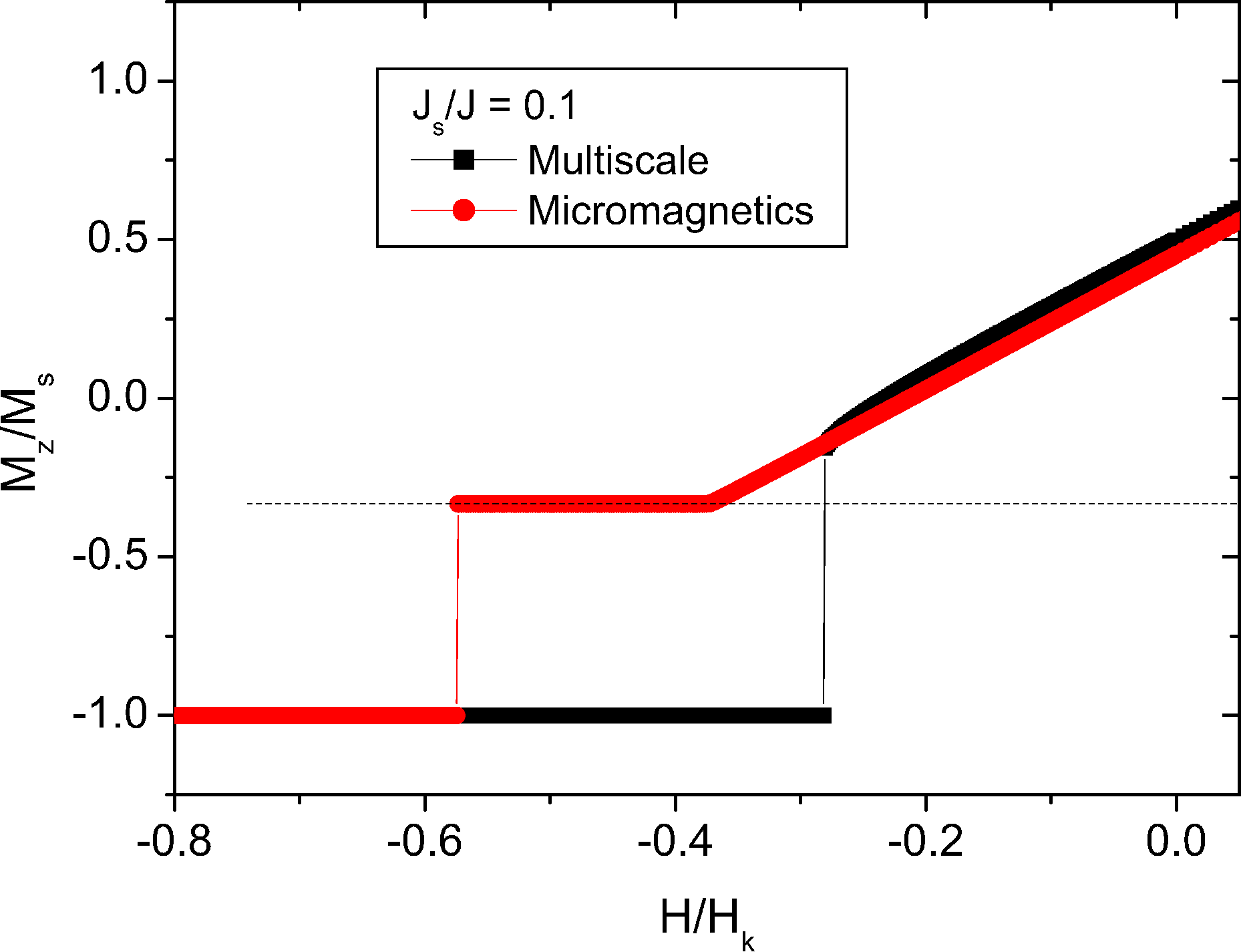Now we will compare the calculations of the micromagnetic model with that of the multiscale model when the interfacial exchange is small. The multiscale model has been already described in Section 2.2.4, but in this case the atomistic region has twice the macromagnetic cell size in each material, giving a thickness of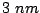treated atomistically on both the FePt and FeRh sides of the interface. The demagnetization curves for interfacial exchange value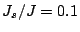are shown in Fig. 3.17. Unlike the one-grain model, the hysteresis cycle of the FeRh part represents a rotation of the magnetization into the easy plane. In this case also an exchange spring is formed at the interface. However, this domain wall represents a magnetization rotation of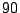degrees rather than a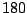degree wall as in the isolated grain. This reversible rotation and the nucleation at positive fields is typical for a hard axis loop. The loops presents a jump corresponding to the depinning of the domain wall and the complete switching of the hard layer. We can observe that, in the multiscale model the complete reversal of the soft layer is not needed to reverse the hard layer. The results of calculations of domain wall structures using the multi-scale model are shown in Fig. 3.18. It can be seen that there is a transition from the discontinuous domain wall structure at low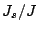to a continuous wall at a critical value of. Forthere is little evolution of the domain wall structure. The predictions of the multiscale model are in marked contrast to those of the micromagnetic approach. This is demonstrated comparing with Fig. 3.19, which shows the domain wall structures calculated using the micromagnetic model. Although the valueis greater than the critical value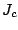predicted by the multi-scale model, the micromagnetic calculation still predicts a discontinuous domain wall. This is because the micromagnetic determination of the exchange energy relies on a long-wavelength approximation, which underestimates the exchange energy associated with rapid spatial variation of the magnetization, thereby allowing discontinuous domain wall structures under conditions where these are not supported by the atomistic calculations. It appears that for the coercivity reduction, a continuous domain wall is necessary, as a consequence, the micromagnetic estimation of the critical exchange value is larger than the atomistic/multiscale estimation. Clearly, a discontinuous domain wall structure, which has an interfacial energy larger than that required to achieve a continuous domain wall, requires a larger field to initiate the propagation of the domain wall leading to magnetization reversal.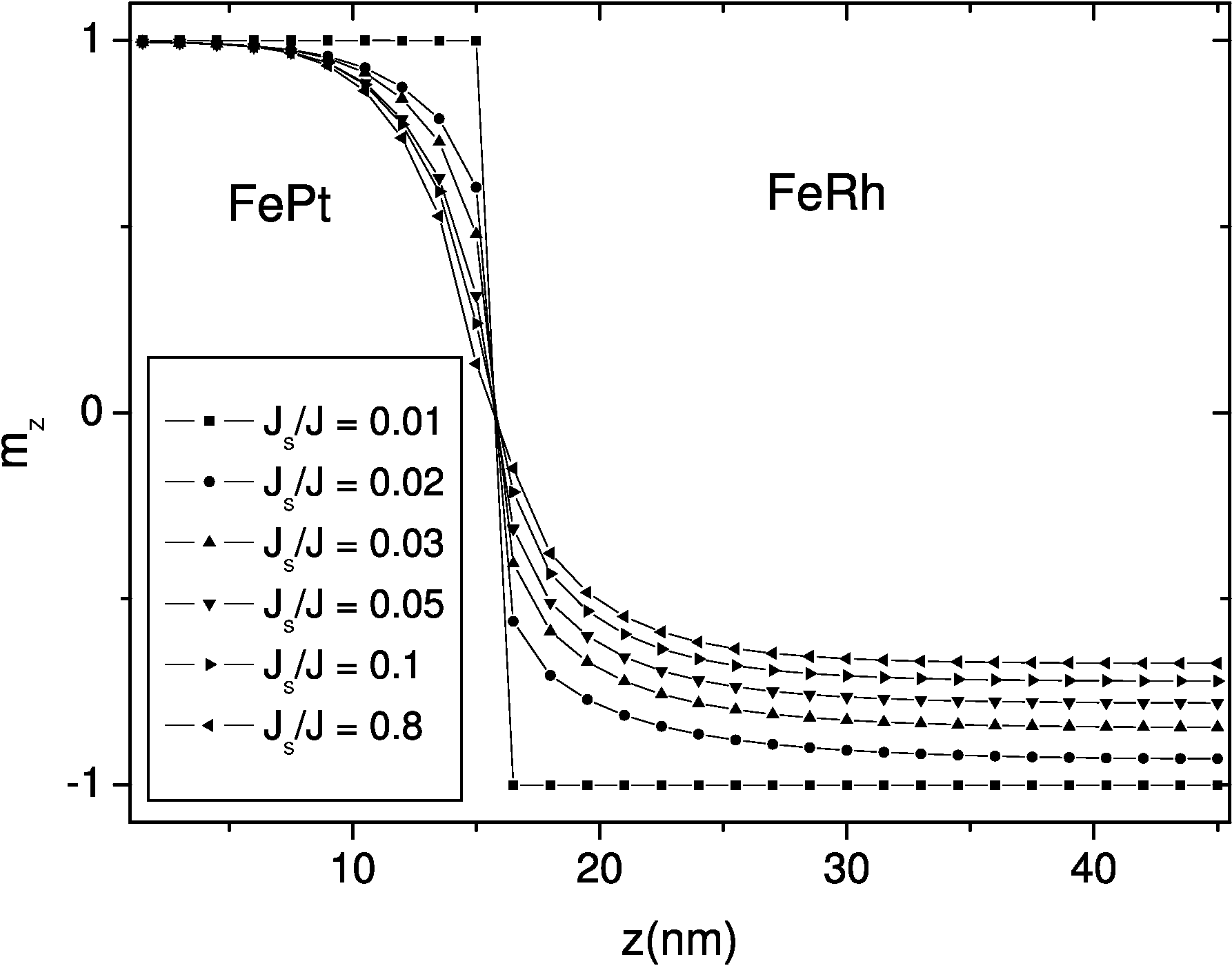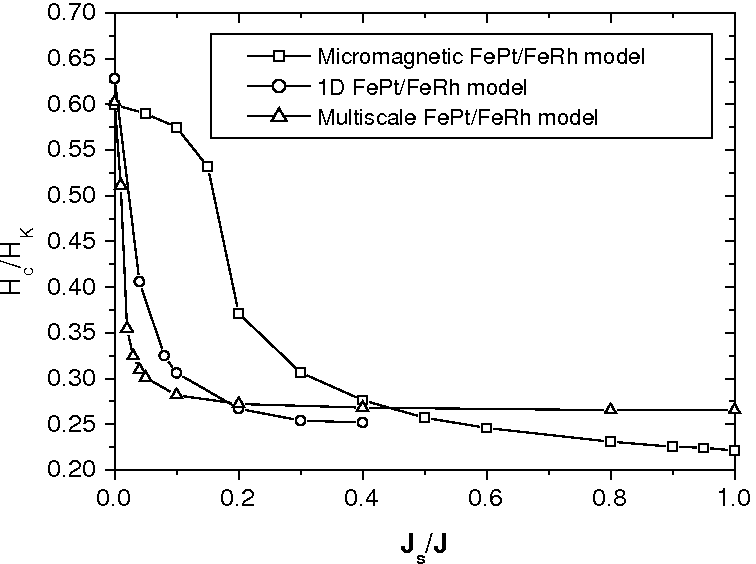These limitations are also responsible for the failure of the micromagnetic model to predict saturation of the coercivity reduction until large values of interfacial exchange. The variation of the coercivity with interfacial exchange energy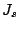for the micromagnetic model is shown in Fig. 3.20. First we discuss the comparison with the 1D'' model. Clearly, the micromagnetic model predictions differ significantly from the 1D'' atomic scale model. It can be seen in Fig. 3.20, the 1D'' model shows saturation in the coercivity reduction at around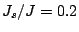, whereas the micromagnetic model predicts a continuous (albeit slow) decrease up to the bulk value of exchange.

We can also compare our micromagnetic results with the analytical expressions of the pinning field Eq. (3.4). In our case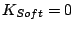yielding a pinning field(cf. the value in Fig. 3.20). For the interfacial exchange value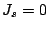the coercive field of the FePt bilayer results from the shape and the magnetocrystalline anisotropies, whose contributions are opposite in sign: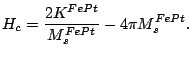(3.6)

Using our model parameters, we obtain the switching field value. Our results from the simulations reasonably agree with these values (see Fig. 3.20).

In conclusion, we have demonstrated that the coercivity reduction in FePt/FeRh bilayers occurs via a complex reversal mechanism involving the penetration of an exchange spring from the soft FeRh into the hard FePt magnetic material. For weak interlayer coupling the standard micromagnetic approach cannot reproduce correctly this mechanism. We should notice here that several authors [Victora 05b] incorporate in their micromagnetic model special boundary conditions resulting from the counting the number of atomistic magnetic moments at the interface. Namely, the interfacial exchange is supposed to be of the form: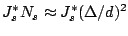(3.7)

where theis the (reduced) atomistic exchange,is the number of exchangely-coupled magnetic moments across the interface,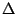- the discretization size (cubic discretization is assumed for simplicity),is the interatomic distance across the interface (cubic lattice is assumed for simplicity). It is clear that this approach gives a different strength of the micromagnetic exchange parameter used at the interface. Since this parameter is in any case phenomenological, the results could be re-scaled. To illustrate this, we have also varied the discretization length in the micromagnetic approach. In this case we also used a square lattice withto simplify calculations. We modeled a grain of, equal thicknesses of FePt and FeRh (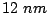) and anisotropy constant. The coercivities obtained for different discretization lengths are shown in comparison with the atomistic simulation in Fig. 3.21. The obtained coercivity presents an increasing reduction with a reducing discretization length, even for values of the discretization length below the lattice size. The fact that the coercivity curves do not saturate to a single curve for discretization lengths below a critical one is a failure of the micromagnetism to describe the soft/hard bilayer. However, all the micromagnetic curves collapse to a single curve if the interfacial exchange is rescalated by the factor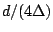, which comes from the comparison of Eq. (2.25) and Eq. (3.7). Although the scaling is successful, it does not allow to make a limit of continuous exchange at the interface, and, therefore, the investigation of the whole range of interfacial exchange parameters. Additionally, this type of approach is essentially a multiscale approach for a step-like interface since it incorporates the atomistic knowledge at the interface assuming that all the parameters variations take place in one interatomic distance.2008-04-04